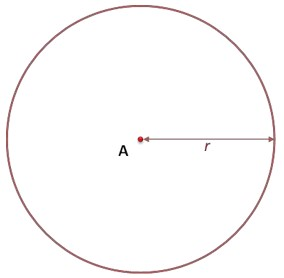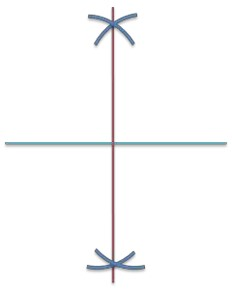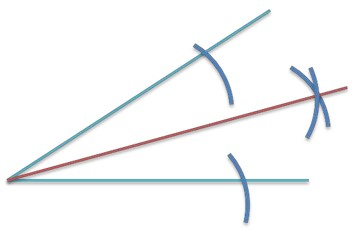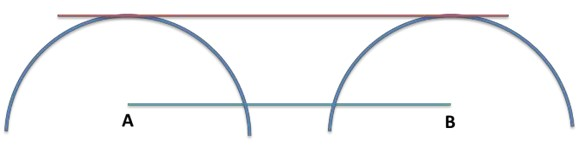4 Ijesha Close, Ilupeju, Lagos
+2348 097 685 118

#### Geometry

##### TRIANGLES• Rectangle: Opposite sides are parallel and equal, all angles 90°. The diagonals bisect each other.• Parallelogram: Opposite sides are parallel and equal, opposite angles are equal, diagonals bisect each other.• Rhombus: is a  parallelogram with all sides equal. Its opposite angles are equal, its diagonals bisect each other.• Trapezium: One pair of its sides are parallel.• Kite: Two pairs of its adjacent sides are equal, its diagonals meet at right angles, bisecting one of them.##### SYMMETRY
• A line of symmetry divides a two-dimensional shape into two congruent (identical) shapes.
• A plane symmetry divides a three-dimensional shape into two congruent solid shapes.
• The number of times a shape fits its outline during a complete revolution is called the order of rotational symmetry.Shape Number of Lines of Symmetry Rotational Symmetry Order
Square 4 4
Rectangle 2 2
Parallelogram 0 2
Rhombus 2 2
Trapezium 0 1
Kite 1 1
Equilateral Triangle 3 3
Regular Hexagon 0 6

• Properties of circles:
• Equal chords are equidistant from the center.
• The perpendicular bisector of a chord passes through the center.
• Tangents from an external point are equal in length.
• Sum of angles at a point = 360°
• Angles on a straight line = 180°
• Sum of angles in a triangle = 180°
• For regular polygons:
• External angles = 360/n
• Sum of interior angles = 180(n – 2)
• Vertically opposite angles (X1-X1, X-X)• Corresponding angles• Alternate angles• Co-interior angles• Exterior angle = Sum of interior angles opposite the exterior angle. (d = a + b)##### CIRCLE THEOREM##### LOCI
• The locus of points equidistant from a point is a circle.• The locus of points equidistant between two points is a perpendicular bisector.• The locus of points equidistant between two lines is an angle bisector.• The locus of points equidistant (along) from a line is a parallel line.